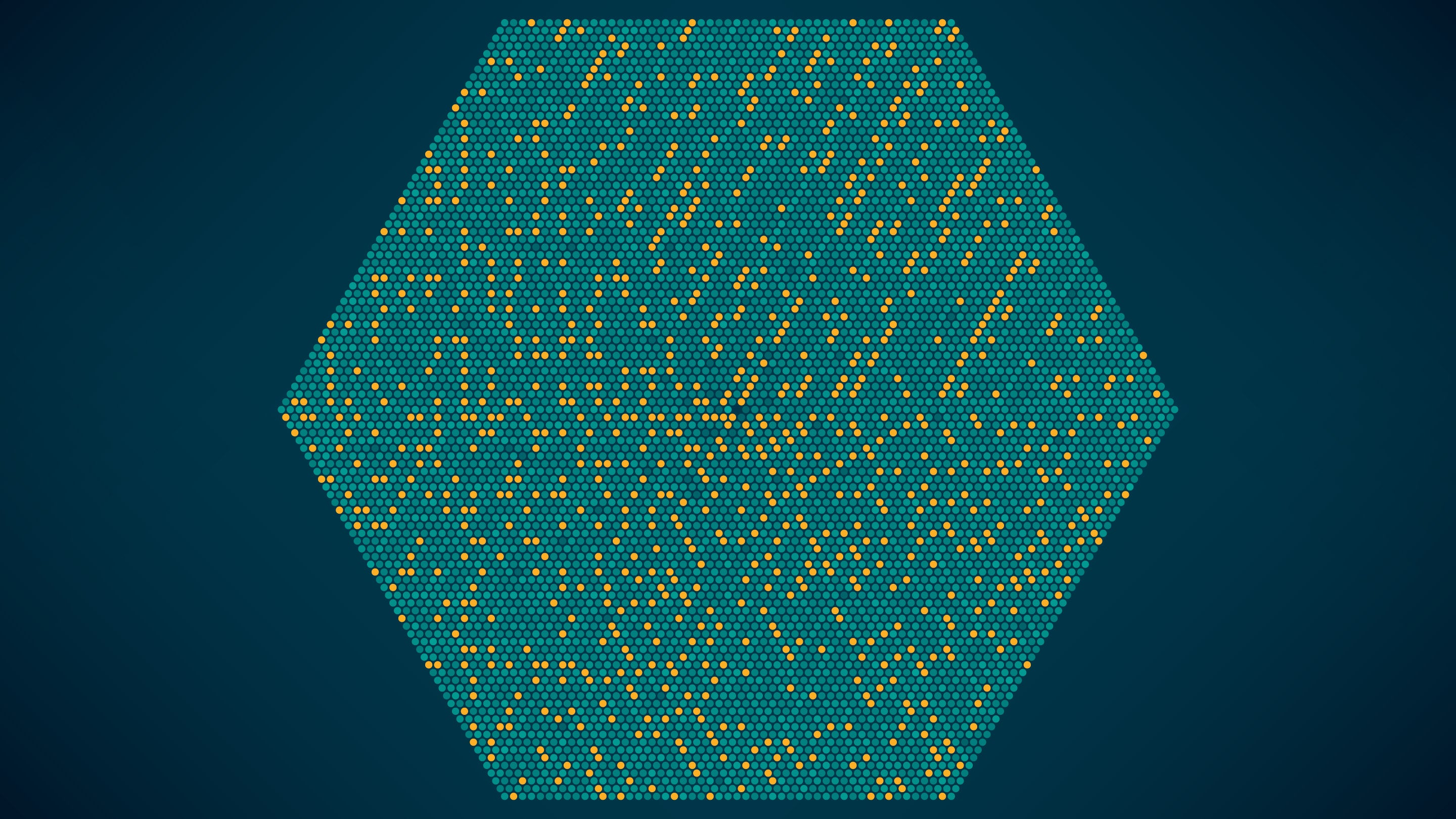# Mathematicians Will Never Stop Proving the Prime Number Theorem

Why do mathematicians enjoy proving the same results in different ways?Explore

“You don’t have to believe in God, but you have to believe in The Book,” the Hungarian mathematician Paul Erdős once said. The Book, which only exists in theory, contains the most elegant proofs of the most important theorems. Erdős’ mandate hints at the motives of mathematicians who continue to search for new proofs of already proved theorems. One favorite is the prime number theorem—a statement that describes the distribution of prime numbers, those whose only divisors are 1 and themselves. While mathematicians never know whether a proof would merit inclusion in The Book, two strong contenders are the first, independent proofs of the prime number theorem in 1896 by Jacques Hadamard and Charles-Jean de la Vallée Poussin.

So what does this theorem actually say?

The prime number theorem provides a way to approximate the number of primes less than or equal to a given number n. This value is called π(n), where π is the “prime counting function.” For example, π(10) = 4 since there are four primes less than or equal to 10 (2, 3, 5 and 7). Similarly, π(100) = 25 , since 25 of the first 100 integers are prime. Among the first 1,000 integers, there are 168 primes, so π(1,000) = 168, and so on. Note that as we considered the first 10, 100 and 1,000 integers, the percentage of primes went from 40% to 25% to 16.8%. These examples suggest, and the prime number theorem confirms, that the density of prime numbers at or below a given number decreases as the number gets larger.

But even if you had an ordered list of positive integers up through, say, 1 trillion, who would want to determine π(1,000,000,000,000) by way of a manual count? The prime number theorem offers a shortcut.

The theorem tells us that π(n) is “asymptotically equal” to n/ln(n), where ln is the natural logarithm. (You can think of an asymptotic equality as an approximate equality, though it is technically more than that.) As an example, let’s estimate the number of primes up to 1 trillion. Instead of counting individual primes to determine π(1,000,000,000,000), you could use this theorem to learn that there are approximately 1,000,000,000,000/ln(1,000,000,000,000) of them, which equals. 36,191,206,825 when rounded to a whole number. This amount is only off by about 4% from the actual answer, 37,607,912,018.

With asymptotic equality, the accuracy improves as you plug larger numbers into the formula. Basically, as you head toward infinity—which is not itself a number, but something larger than any number—the approximate equality in the theorem approaches an actual equality. This is despite the fact that the actual number of primes will always equal an integer, while on the other side of the asymptotic equality, the fraction involving the natural logarithm function could equal any value on the real number line. This connection between integers and real numbers is counterintuitive at best.

It’s mind-blowing stuff, even among mathematicians. Maddeningly, the statement of the prime number theorem does not hint at why any of this is true.

“The theorem was never about the theorem. It was always about the proof,” says Michael Bode of the Queensland University of Technology.

“The theorem was never about the theorem. It was always about the proof,” said Michael Bode, a mathematics professor at Queensland University of Technology in Australia.

As elegant as they were, Hadamard’s and de la Vallée Poussin’s original proofs relied on complex analysis—the study of functions with imaginary numbers—which some found unsatisfying as the statement of this theorem does not itself involve complex numbers. However, G.H. Hardy, in 1921, dubbed the prospect of a nonanalytic proof—known as an elementary proof—of the prime number theorem “extraordinarily unlikely” and claimed that if anyone should find one, it would call for “theory to be rewritten.”

Atle Selberg and Erdős himself took on the challenge, and in 1948 they each published new, independent elementary proofs of the prime number theorem using properties of logarithms. These proofs enticed other mathematicians to consider similar methods for number theory conjectures previously considered too profound for such seemingly simple methods. Many exciting results followed, including Helmut Maier’s 1985 elementary proof showing unexpected irregularities in the distribution of primes.

“So many open questions build on the prime number theorem,” said Florian Richter, a mathematician at Northwestern University who posted a new elementary proof of this celebrated statement. Richter found his proof while trying to prove a far-reaching extension of the prime number theorem.

So many open questions build on the prime number theorem, says Florian Richter of Northwestern University.

Over time, number theorists helped establish a culture in which mathematicians worked on proving and re-proving theorems not just to verify statements, but also to improve their skills at proving theorems and their understanding of the math involved.

This goes beyond the prime number theorem. Paulo Ribenboim cataloged at least 7 proofs of the infinitude of primes. Steven Kifowit and Terra Stamps identified 20 proofs demonstrating that the harmonic series, 1+ 1/2 + 1/3 + 1/4 + 1/5 + 1/6 + 1/7 + …, does not equal a finite number, and Kifowit later followed up with 28 more. Bruce Ratner cites more than 371 different proofs of the Pythagorean theorem, including some gems provided by Euclid, Leonardo da Vinci and U.S. President James Garfield, who was a member of Congress from Ohio at the time.

This habit of re-proving things is now so ingrained, mathematicians can literally count on it. Tom Edgar and Yajun An noted that there have been 246 proofs of a statement known as the quadratic reciprocity law following Gauss’ original proof in 1796. Plotting the number of proofs over time, they extrapolated that we could expect the 300th proof of this theorem around the year 2050.

“I love new proofs of old theorems for the same reason I love new roads and shortcuts to places I’ve already been,” said Sophia Restad, a graduate student at Kansas State University. These new paths provide mathematicians with a figurative sense of place for intellectual activity.

Mathematicians may never stop searching for new and more illuminating paths to the prime number theorem and other
beloved theorems. With luck, some of them will even merit
inclusion in The Book.Enjoy unlimited Nautilus articles, ad-free, for as little as \$4.92/month.